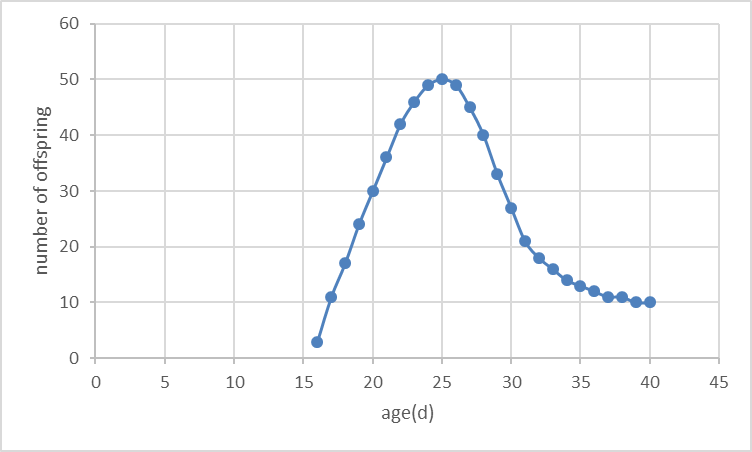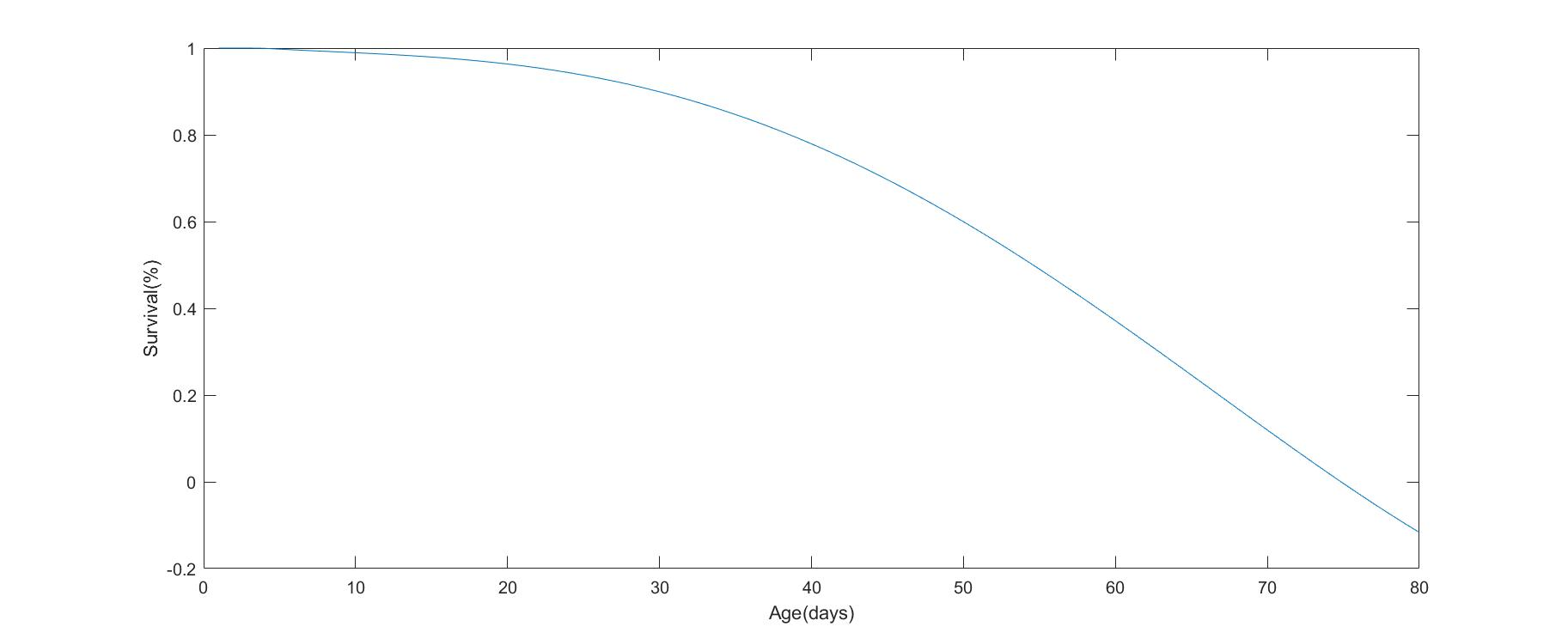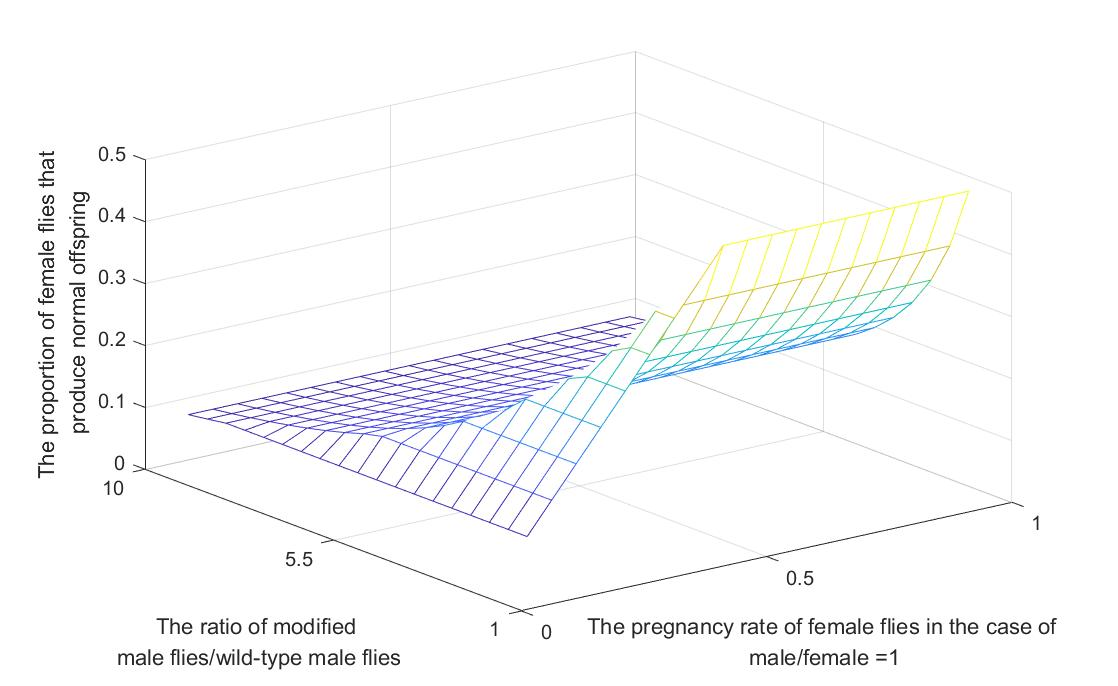# Team:Tongji China/Model

Tongji iGEM - Model
Tongji iGEM
TongJi iGEM
Model
Modelling allows us to predict what is too complex to be determined experimentally at this stage.
expand_more

#### Background

Male-male courtship will undoubtedly affect the population growth. We believe that the method of releasing male-male courtship fruit flies can be used for population control, and be further extended to controlling more kinds of pest. We also found that the "sterilization" of mosquitoes, which do not hatch eggs, has been researched for population control. We think that the two aproaches may work more effectively when used together, so we built a mathematical model to validate our idea.

#### Theory

First, we set the program with the thoughts of "sterilization" mosquitoes as a1. We can assume that the number of male mosquitoes and female mosquitoes in the wild environment is set to 1, and the number of sterilized male mosquitoes is 5, so the number of offspring is reduced to 1/6. With the universality hypothesis, the number of wild-type male mosquitoes and female mosquitoes in the environment is 1, and the amount of sterilized male mosquitoes is y, that is, the sterilized male mosquitoes released are as much as y times of the wild-type mosquitoes, we will use this parameter later. Then set that the proportion of all survivors as p(a1), and we can get: $$p(a_1) = \frac{1}{1+y} \qquad $$ Therefore we tried to apply this idea to our project, to make our modified fruit flies not only having the pursuit of the same characteristics but also making the next generations which cannot be hatched. We set this program as '''a1'''. Assuming that the number of wild-type male and female fruit flies in the environment is 1, the amount of fruit fly is converted to y. According to the results of the behavioral experiments, the modified fruit flies have the same probabilities to male and female, The ratio of fruit flies to normal hatching p(a1) is: $$p(a_2) = \frac{1}{1+\frac{y}{x}} \qquad $$ It can be found that the "sterilization" individuals in previous projects are superior to our male-male courtship individuals in terms of the inhibition of the number of offspring populations. However, in our model, each female flies are mated when the ratio of male to female is at 1: 1. Suppose the mating rate is k, so k=1. But k should be, in the natural situation, less than 1. When increasing the number of male fruit flies, the ratio k of mating female flies to the total number of females increased. It changes into k’: $$k' = k \cdot (1+y) \qquad $$ But we should make a discussion of this formula. When k*(1+y)>1, we make k’=1; when k*(1+y)<=1, making k’=k*(1+y). $$k'= \begin{cases} k \cdot (1+y) \qquad k \cdot (1+y) \le 1 \\ 1 \quad \qquad \qquad k \cdot (1+y) \gt 1 \\ \end{cases} \qquad $$ So p(a1) can be worked out: $$p(a_1)= \begin{cases} k \qquad \qquad k \cdot (1+y) \le 1 \\ \frac{1}{1+y} \quad \qquad k \cdot (1+y) \gt 1 \\ \end{cases} \qquad $$ In our project’s model, we assume that among the male fruit flies with the number of y, half sterile male flies that have male-male courtship, and the number of modified flies who chases modified flies is x. And we can also assume that half of these flies are active. And among the sterile flies with male-male courtship, the number of flies who is active is (y/2-x). The pursuer the two different flies chose is (y/2-x/2) and (1+y/2) respectively. So we can conclude a equation as follow $$\frac {\frac{x}{2}} {\frac{y}{2}-x} = \frac {\frac{y}{2}-\frac{x}{2}} {1+\frac{y}{2}} \qquad $$ By Solving equation, we can know that: $$x = \frac {2y+1- \sqrt{2y^2+4y+1}} {2} \qquad $$ After release sterile male flies with male-male courtship, the ratio of mated female flies changes to: $$k' = \frac {k \cdot (1+ \frac{y}{2})} {1+ \frac{y}{2}-x} \qquad $$ So p(a1) can be worked out: $$p(a_2)= \begin{cases} \frac{k}{1+ \frac{y}{2}-x} \quad \qquad \frac{k \cdot (1+ \frac{y}{2})}{1+ \frac{y}{2}-x} \le 1 \\ \frac{1}{1+ \frac{y}{2}} \qquad \qquad \frac{k \cdot (1+ \frac{y}{2})}{1+ \frac{y}{2}-x} \gt 1 \\ \end{cases} \qquad $$ As we can see, although the radio of offspring that was incubated normally increased a little than previous research, which is a disadvantage in population control, but if we regard the female flies’ crossover rate less then 1, among the offspring of sterile flies with male-male courtship, the number of flies that can be incubated normally will decrease, which can control the population in to some extent. And the effect of this way is better than releasing sterile male flies.

As simulation the change of population in one day is not enough to prove that this way can control the population. So we decided to simulation the change of population in fifteen day. In order to achieve this goal, we will use Leslie matrix.
Leslie Matrix
In 1945, Leslie P H. introduced a mathematical method to predict the age structure and population through time from the age structure of the initial population.

Depending on the physiological characteristics of each individual, the maximum life age is divided into M groups. The objective it to find how the age distribution evolves. The time starts from t=0 and evolves in steps (t=0, 1, 2...), and the interval is the same as that of the age group.

Suppose that, at the beginning (t=0), the number of individuals in the I age group was ni(0), i= 1, 2,... m. so the age distribution vector is: $$\overrightarrow n(0) = [n_1(0), n_2(0), …, n_m(0)]^T$$ The reproductive rate of the I age group is fi(0) ,i= 1, 2,... M;
The survival rate is Si(>0), i= 1, 2,... m - 1;
Between two periods, there is an iterative relationship between the number of individuals in each age group ni: $$\begin{cases} n_1 (t + 1) = \sum_{i=1}^m f_i \cdot n_i(t) = f_i \cdot n_1 (t) + f_2 \cdot n_2(t) + … + f_m \cdot n_m (t) \\ n_i (t + 1) = S_{i-1} \cdot n_{i-1}(t) \qquad i = 2, 3, …,m \\ \end{cases}$$ Note 1: In fi new individuals who have been born within the period of t but have not lived to the (t+ 1) period have been deducted.
Note 2: Usually in the population of bisexual reproduction, only the number of females is counted.

In matrix form: $$L=\begin{bmatrix}{} f_1 & f_2 & ... & f_{m-1} & f_m\\ S_1 & 0 & 0 & 0 & 0\\ 0 & S_2 & 0 & 0 & 0\\ 0 & 0 & ... & 0 & 0\\ 0 & 0 & 0 & S_{m-1} & 0 \end{bmatrix}$$ It's called the Leslie matrix.
Since $$\overrightarrow n(t) = [n_1(t), n_2(t), …, n_m(t)]^T$$ , then equation  can be expressed as $$\overrightarrow n(t+1) = \overrightarrow n(t+1) L$$ So if L and n(0) are known, for any t>0: $$\overrightarrow n(t) = \overrightarrow n(0) L^t$$ After reviewing the literature and doing our own experiments, we assume the life structure of fruit flies as:

During adulthood fruit flies have desire to court others and can be pregnant and the probability won't change with age.
During elderhood fruit flies can barely attract others and barely breed offsprings, so we assume the fertility rate and the survival rate are zero in the Lesli matrix.

Based on the structure, we obtained the curves of fertility and survival.

Fertility curve:Survival curve:#### Results and Discussion

1. In a1, the results of p are as follow:Figure 1 [raw data]

2. In a1, the results of p are as follow:Figure 2 [raw data]

3. The results of p(a1)-p(a1) are as follow:Figure 3 [raw data]

It can be seen that in the case of the small mating rate, the inhibition effect of releasing sterile male flies with male-male courtship is more obvious to reducing population quantity, which is consistent with the purpose of our modeling.

4. y=5, the effect of different mating rates k on the results of p in the two methods is as follows:Figure 4

In the figure above, the yellow line represents the sterile male flies with male-male courtship, and the purple line represents the sterile male flies. We can see that when the mating rate is less than 0.7, releasing sterile male flies with male-male courtship cause more obvious effect.

5. y=5, the effect of different mating rates k on the final population in 15 days is as follows:Figure 5 - The mating rate is 0.1Figure 6 - The mating rate is 0.2Figure 7 - The mating rate is 0.3Figure 8 - The mating rate is 0.4Figure 9 - The mating rate is 0.5Figure 10 - The mating rate is 0.6Figure 11 - The mating rate is 0.7Figure 12 - The mating rate is 0.8Figure 13 - The mating rate is 0.9Figure 14 - The mating rate is 1.0

In the figure above, the blue line represents the number of populations that have been added to the sterile male flies with male-male courtship, and the red line represents the number of populations that have been added to the sterile male flies. By comparison, the number of colonies after the addition of the sterile male flies with male-male courtship was lower in 15 days, as long as the mating rate was below 0.5.

Discussion

Although we get the curve of the propotion of female flies that can profuce normal offsprings under different y and k, we don't have the marginal cost curve. So we can't figure out the best y under different k to control the population number in the most cost-effective way. It will be a hard and time-costing work to do, but it will be necessary if we wan't to put it into use.
Ignis Fly

Tongji_China iGEM 2017 Team
Human Practiceschevron_right